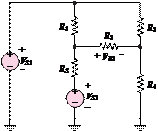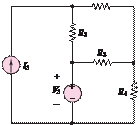### Create an Account

Already have account?

### Forgot Your Password ?

Home / Questions / In the circuit in Figure P321 assume the source voltage and source current and all resista...

# In the circuit in Figure P321 assume the source voltage and source current and all resistances are known a Write the node equations required to determine the Figure

In the circuit in Figure P3.21, assume the source voltage and source current and all resistances are known.

a.    Write the node equations required to determine the

Figure P3.22

3.23  In the circuit shown in Figure P3.23, VS2  and RS

model a temperature sensor, that is,

VS2  = kT                  k = 10 V/◦C

VS1  = 24 V            RS  R1  = 12 kQ

R2  = 3 kQ         R3  = 10 kQ

node voltages.

b.    Write the matrix solution for each node voltage in

R4  = 24 kQ      VR3

= −2.524 V

terms of the known parameters.

The voltage across R3, which is given, indicates the temperature. Determine the temperature.R1Figure P3.21

Jun 19 2020 View more View Less

#### Answer (Solved)Subscribe To Get Solution